#### You may also like### Three Cubes

Can you work out the dimensions of the three cubes?### F'arc'tion

At the corner of the cube circular arcs are drawn and the area enclosed shaded. What fraction of the surface area of the cube is shaded? Try working out the answer without recourse to pencil and paper.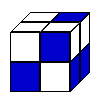### Cubic Conundrum

Which of the following cubes can be made from these nets?

# Peeling the Apple or the Cone That Lost Its Head

##### Age 14 to 16 Challenge Level:

Question : If this sector had a radius of 10 units what would the area be?

I need to calculate $\frac{3}{4}$ of the whole circle : $\frac{3}{4}$ of $\pi$ times $10^2$ is 236 sq.units correct to 3 significant figures

Question : If the sector is made into a cone by closing up the gap, what will be the base radius for that cone?

$\frac{3}{4}$ of the full circumference of the large incomplete circle is used to make the complete circumference of the cone base.

I then realised that it isn't actually necessary to calculate these lengths.

For every circle the diameter and circumference keep their ratio to each other (that's what $\pi$ is) so the new diameter is just $\frac{3}{4}$ of the old diameter, because the new circumference is $\frac{3}{4}$ of the old circumference, so the cone's base radius is 7.5 units.

Question : If the cone now suffers a horizontal cut which removes its top, reducing its height by half, how much curved surface area remains?

Think of it like this :The original whole sector now has its centre missing.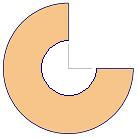The original and the removed sector are similar shapes with a line ratio of 1:2

So the area of the original sector must be four times the area of the removed sector (that's just squaring the line ratio) which means that the curved surface area of the lower remaining part of the cone is $\frac{3}{4}$ of the sector already calculated.

And that comes to 177 sq.units (3 sf)

Dean from Cheadle & Marple College used reasoning like this to get a general formula for the area of a whole cone's curved surface :

Starting with a cone whose base radius is r and slope length is s. The curved surface is a sector from a circle with radius s.

The sector fraction is found by comparing the cone base circumference with the circumference of a circle whose radius is s.

So the fraction is r/s ,the whole area was $\pi$ $s^2$ and that simplifies to $\pi$sr for a cone's surface area not including the base.

Dean then produced a general formula for the area of a frustum's curved surface.

R and r are the radii top and bottom for the frustum and s is the slope length.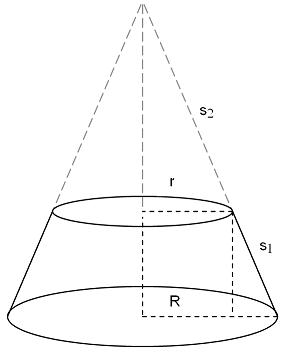This involves some fiddling with the algebra but it's a nice result when you have done it.

What's needed is the difference in surface area between the two cones.

From the diagram you can see that a length $s_2$ will be involved which isn't very convenient because $s_2$ isn't a length you can measure somewhere on the frustum.

However if you notice the similar triangles present in the diagram you can find a connection between $s_1$, $s_2$, r and R

And from that $s_2$ can be expressed as a formula using only $s_1$, r and R

This formula can replace any $s_2$ in the area expression and when you tidy things up (not that quick to do) it reduces to the pleasantly simple $\pi$ s(r+R)

Next Dean explained that when the apple (sphere) is approximated to a stack of frustums (he used five) you can find all the lengths you need so that you can use that formula for the area of the curved surface on each frustum in turn, then for m a total.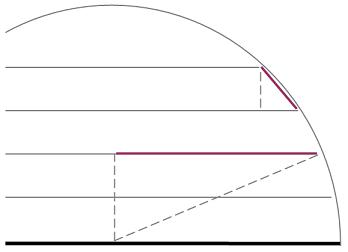If you know how thick you've chosen the frustums to be you know the height.

The radius of the sphere you know and so applying Pythagoras' Theorem it is possible to find any radii lengths required.

Using Pythagoras also to find the slope lengths each time by using the thickness of the frustum and the difference between the top and bottom radii.

Here's part of Dean's spreadsheet and his comment on the result.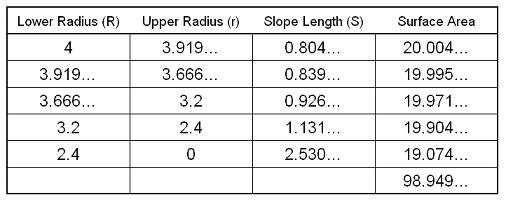Thus the amount of peel of an apple of diameter 8cm is 197.899 (3sf) after doubling the hemisphere result from the sheet.

If this answer is compared to the result from using the equation for surface area of a sphere which is 4 $\pi$ $r^2$ and gives 201.062 . . . it can be seen that there is a percentage error of 1.57%.

If 50 frustums are used the percentage error drops to 0.02%.

Which leaves us pleasantly curious about that formula Dean found which calculates the surface area for a sphere.

If I know the surface area for a sphere of unit radius I can say that the surface area for a sphere of radius r would be $r^2$ times as much so it's the right kind of formula. I wonder if we can verify that 4$\pi$ multiplier.

Continuing that line of thinking. If I know the volume for a sphere of unit radius I can say that the volume for a sphere of radius r would be $r^3$ times as much. That tells me what type of formula to expect now perhaps I can use that spreadsheet technique to find the volume of a sphere with unit radius. I wonder if it's a nice number of $\pi$ like the surface area?